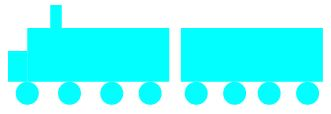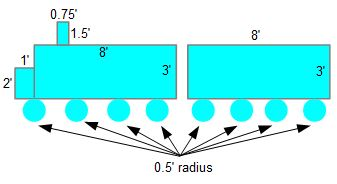# Using Geometry to Analyze Math Problems

Instructor: Yuanxin (Amy) Yang Alcocer

Amy has a master's degree in secondary education and has taught math at a public charter high school.

As you learn more math concepts, it helps you solve problems faster and easier. This is the case when it comes to using geometry to solve math problems. Read this lesson to learn how common geometric shapes can help you solve problems.

## A Math Problem

Geometry is the field of mathematics that deals with points, lines, and shapes. Once you've learned some geometry, you'll be able to solve many real world problems. Geometry skills have proven useful for millennia. For example, the Egyptians used geometry to help them build the pyramids, which are still standing today. In this lesson, you'll learn how you can use geometry skills to help you solve problems.

## Using Geometry

Let's see how you can use geometry to help you solve this math problem.

Thomas wants to paint a train onto the wall of his daughter's room. He has the shape of the train outlined already. Now he needs to figure out how much paint he needs to purchase to paint the train. So, he needs to calculate the area of his train shape. Here is what his train looks like.The way Thomas can use geometry to help him find his answer is by separating his train shape into simple, distinct, geometric shapes and then use the area formulas associated with those shapes. The shapes in his train include rectangles and circles. Right now, all Thomas has is an outline and the measurements of each segment of his outline. To help him find the total area, he splits his shape into a number of circles and rectangles and writes the measurements on each side and radius.By splitting your math problem into easily recognizable geometric shapes, you can then proceed to use the formulas and properties associated with the shapes to arrive at your solution.

To solve Thomas' problem, he can now find the area of each geometric shape and then add them all up to find the total area that needs to be painted.

Looking at his train separated into separate shapes, Thomas sees that he has 4 rectangles and 8 circles. He remembers that the formula to find the area of a rectangle is the length times the width. For circles, the formula for area is pi times the square of the radius.

Making these calculations, Thomas gets these numbers:

• For the rectangle in the front of the train: 1 * 2 = 2 square feet.
• For the little rectangle on the top of the train: 1.5 * 0.75 = 1.125 square feet.
• For the big rectangle making up the first section of the train: 8 * 3 = 24 square feet.
• For the big rectangle making up the second section of the train: 8 * 3 = 24 square feet.
• For the 8 circles making up the wheels: 3.14 * 0.52 * 8 = 6.28 square feet.

Adding all these numbers up, Thomas gets a total area of 2 + 1.125 + 24 + 24 + 6.28 = 57.405 square feet.

The key to solving math problems with the help of geometry is to divide your problem into recognizable geometric shapes that are easy to work with. After you've done that, then you can use the formulas related to those shapes to solve your problem.

To unlock this lesson you must be a Study.com Member.

### Register to view this lesson

Are you a student or a teacher?

### Unlock Your Education

#### See for yourself why 30 million people use Study.com

##### Become a Study.com member and start learning now.
Back
What teachers are saying about Study.com

### Earning College Credit

Did you know… We have over 160 college courses that prepare you to earn credit by exam that is accepted by over 1,500 colleges and universities. You can test out of the first two years of college and save thousands off your degree. Anyone can earn credit-by-exam regardless of age or education level.